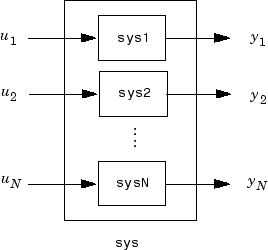# append

Group models by appending their inputs and outputs

## Syntax

```sys = append(sys1,sys2,...,sysN) ```

## Description

`sys = append(sys1,sys2,...,sysN)` appends the inputs and outputs of the models `sys1`,...,`sysN` to form the augmented model `sys` depicted below.For systems with transfer functions H1(s), . . . , HN(s), the resulting system `sys` has the block-diagonal transfer function

`$\left[\begin{array}{cccc}{H}_{1}\left(s\right)& 0& \dots & 0\\ 0& {H}_{2}\left(s\right)& \cdots & ⋮\\ ⋮& ⋮& \ddots & 0\\ 0& \cdots & 0& {H}_{N}\left(s\right)\end{array}\right]$`

For state-space models `sys1` and `sys2` with data (A1B1C1D1) and (A2B2C2D2), `append(sys1,sys2)` produces the following state-space model:

`$\begin{array}{c}\left[\begin{array}{c}{\stackrel{˙}{x}}_{1}\\ {\stackrel{˙}{x}}_{2}\end{array}\right]=\left[\begin{array}{cc}{A}_{1}& 0\\ 0& {A}_{2}\end{array}\right]\left[\begin{array}{c}{x}_{1}\\ {x}_{2}\end{array}\right]+\left[\begin{array}{cc}{B}_{1}& 0\\ 0& {B}_{2}\end{array}\right]\left[\begin{array}{c}{u}_{1}\\ {u}_{2}\end{array}\right]\\ \left[\begin{array}{c}{y}_{1}\\ {y}_{2}\end{array}\right]=\left[\begin{array}{cc}{C}_{1}& 0\\ 0& {C}_{2}\end{array}\right]\left[\begin{array}{c}{x}_{1}\\ {x}_{2}\end{array}\right]+\left[\begin{array}{cc}{D}_{1}& 0\\ 0& {D}_{2}\end{array}\right]\left[\begin{array}{c}{u}_{1}\\ {u}_{2}\end{array}\right]\end{array}$`

## Arguments

The input arguments `sys1`,..., `sysN` can be model objects s of any type. Regular matrices are also accepted as a representation of static gains, but there should be at least one model in the input list. The models should be either all continuous, or all discrete with the same sample time. When appending models of different types, the resulting type is determined by the precedence rules (see Rules That Determine Model Type for details).

There is no limitation on the number of inputs.

## Examples

collapse all

Create a SISO transfer function.

```sys1 = tf(1,[1 0]); size(sys1)```
```Transfer function with 1 outputs and 1 inputs. ```

Create a SISO continuous-time state-space model.

```sys2 = ss(1,2,3,4); size(sys2)```
```State-space model with 1 outputs, 1 inputs, and 1 states. ```

Append the inputs and outputs of `sys1`, a SISO static gain system, and `sys2`. The resulting model should be a 3-input, 3-output state-space model.

`sys = append(sys1,10,sys2)`
```sys = A = x1 x2 x1 0 0 x2 0 1 B = u1 u2 u3 x1 1 0 0 x2 0 0 2 C = x1 x2 y1 1 0 y2 0 0 y3 0 3 D = u1 u2 u3 y1 0 0 0 y2 0 10 0 y3 0 0 4 Continuous-time state-space model. ```
`size(sys)`
```State-space model with 3 outputs, 3 inputs, and 2 states. ```

## Version History

Introduced before R2006a